Miscellaneous

Chapter 11 Class 12 Three Dimensional Geometry
Serial order wise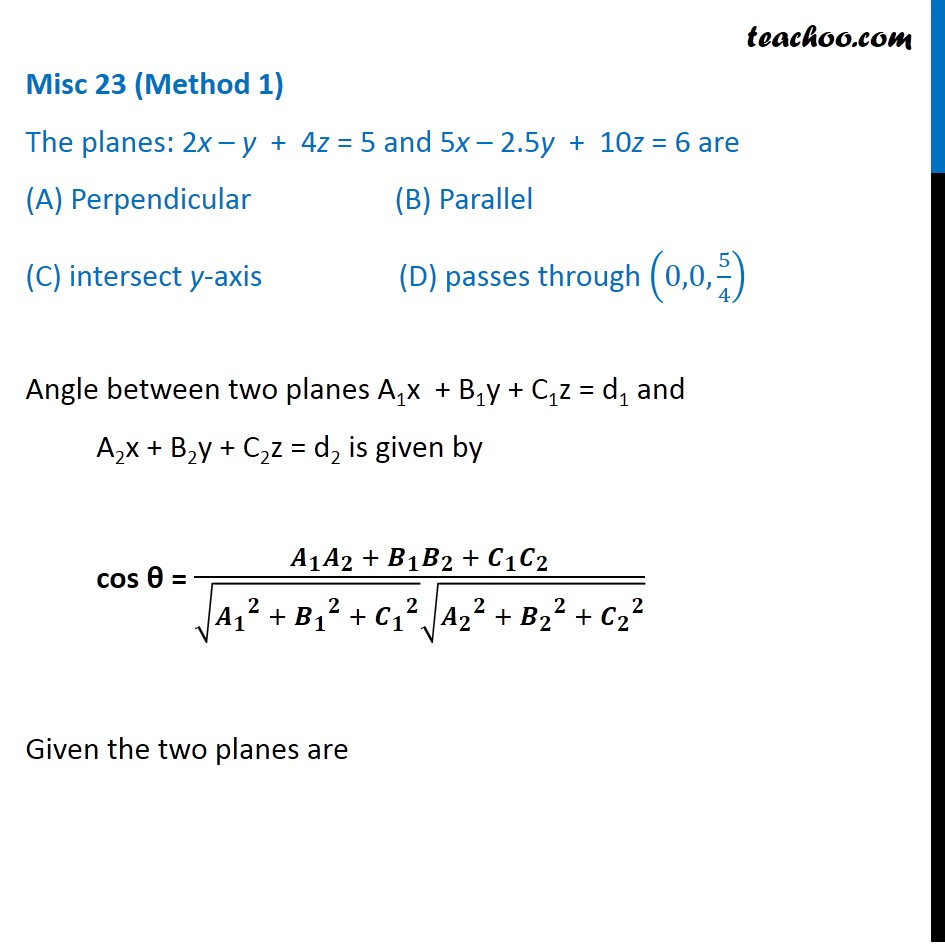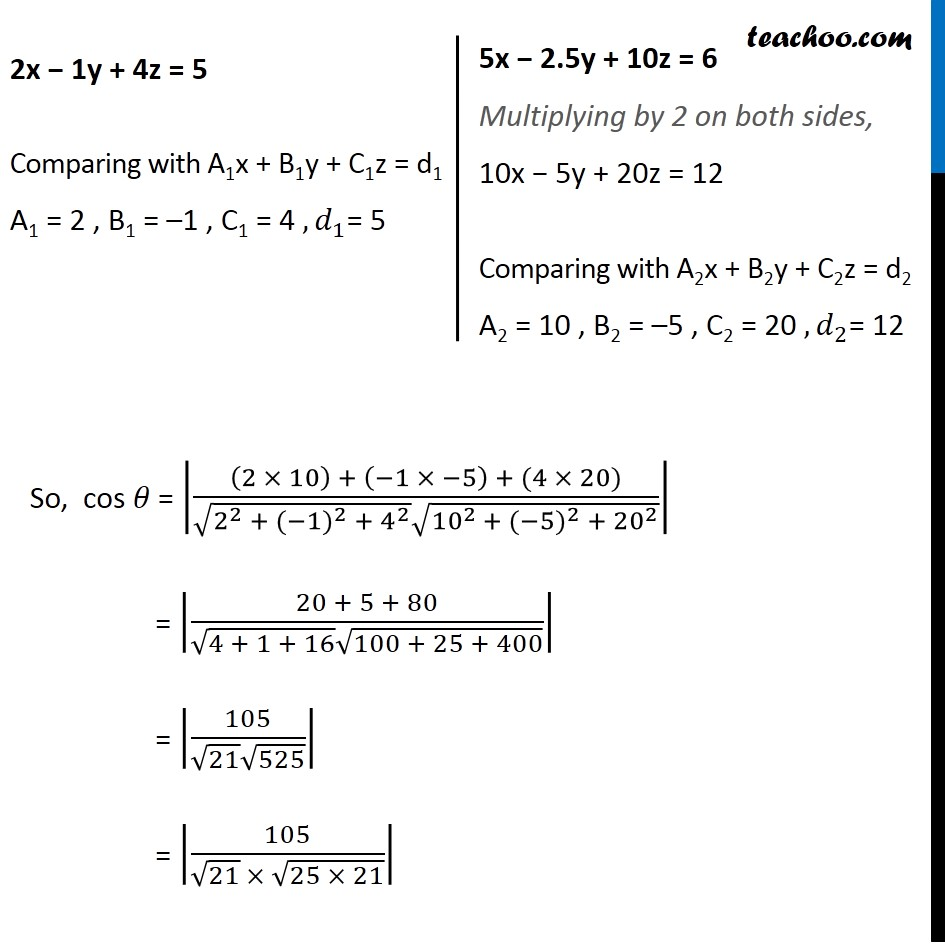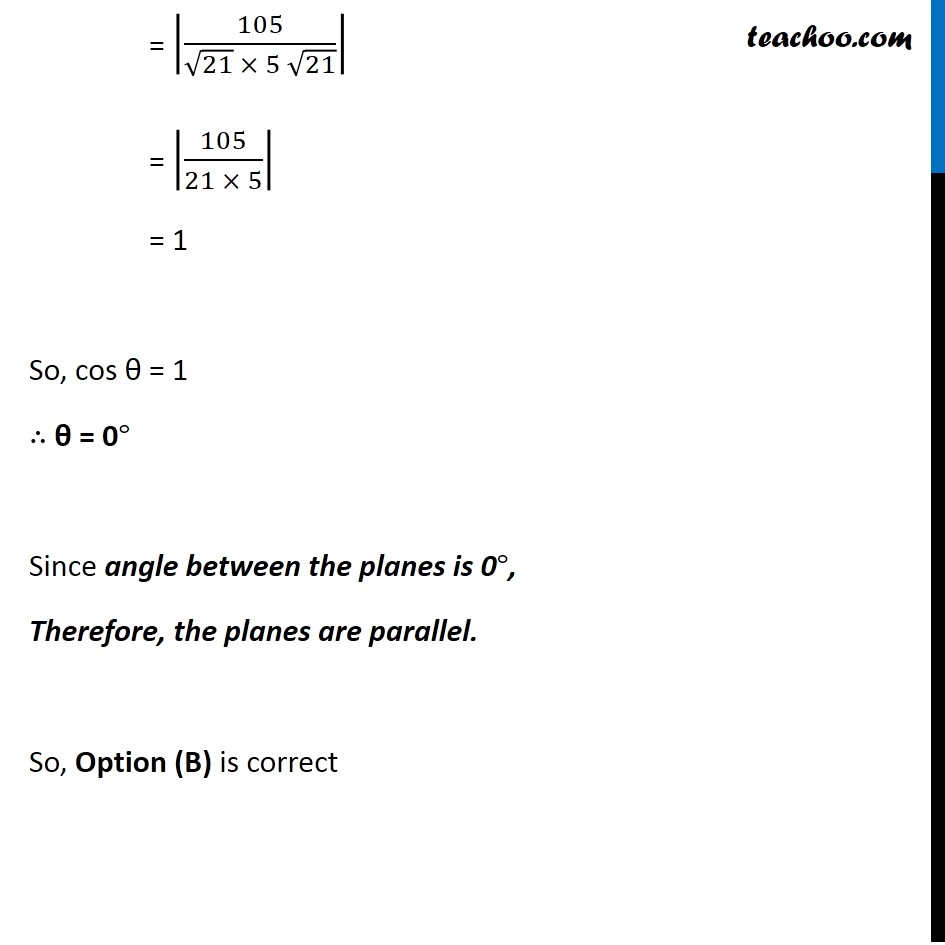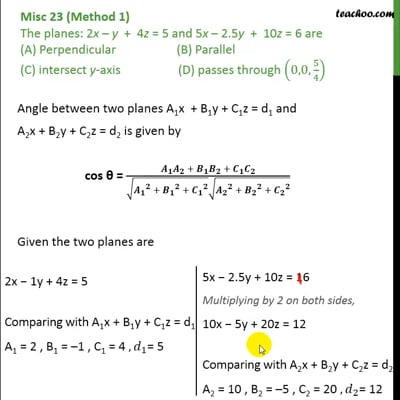This video is only available for Teachoo black users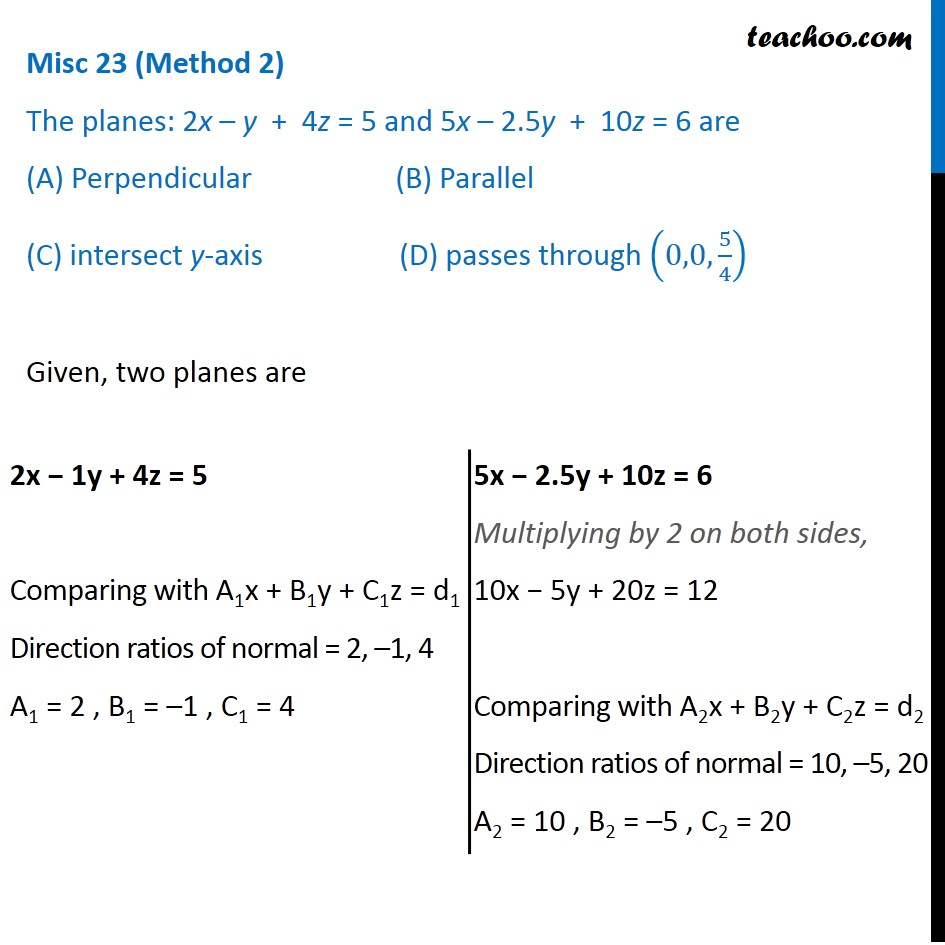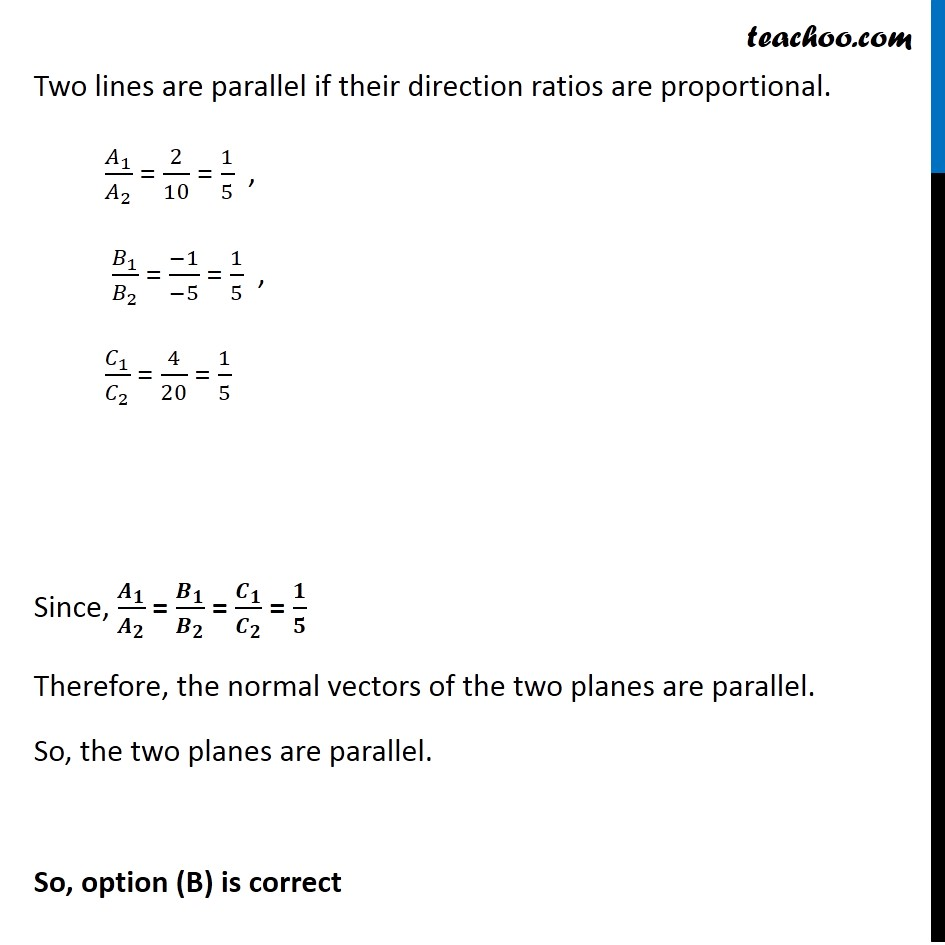This video is only available for Teachoo black users

Introducing your new favourite teacher - Teachoo Black, at only ₹83 per month

### Transcript

Misc 23 (Method 1) The planes: 2x – y + 4z = 5 and 5x – 2.5y + 10z = 6 are (A) Perpendicular (B) Parallel (C) intersect y-axis (D) passes through (0,0, 5/4) Angle between two planes A1x + B1y + C1z = d1 and A2x + B2y + C2z = d2 is given by cos θ = (𝑨_𝟏 𝑨_𝟐 + 𝑩_𝟏 𝑩_𝟐 + 𝑪_𝟏 𝑪_𝟐)/(√(〖𝑨_𝟏〗^𝟐 + 〖𝑩_𝟏〗^𝟐 + 〖𝑪_𝟏〗^𝟐 ) √(〖𝑨_𝟐〗^𝟐 + 〖𝑩_𝟐〗^𝟐 + 〖𝑪_𝟐〗^𝟐 )) Given the two planes are 2x − 1y + 4z = 5 Comparing with A1x + B1y + C1z = d1 A1 = 2 , B1 = –1 , C1 = 4 , 𝑑_1= 5 5x − 2.5y + 10z = 6 Multiplying by 2 on both sides, 10x − 5y + 20z = 12 Comparing with A2x + B2y + C2z = d2 A2 = 10 , B2 = –5 , C2 = 20 , 𝑑_2= 12 So, cos 𝜃 = |((2 × 10) + (−1 × −5) + (4 × 20))/(√(2^2 + (〖−1)〗^2 + 4^2 ) √(〖10〗^(2 )+ (〖−5)〗^2 + 〖20〗^2 ))| = |(20 + 5 + 80)/(√(4 + 1 + 16) √(100 + 25 + 400))| = |105/(√21 √525)| = |105/(√21 × √(25 × 21))| = |105/(√21 × 5 √21)| = |105/(21 × 5)| = 1 So, cos θ = 1 ∴ θ = 0° Since angle between the planes is 0°, Therefore, the planes are parallel. So, Option (B) is correct Misc 23 (Method 2) The planes: 2x – y + 4z = 5 and 5x – 2.5y + 10z = 6 are (A) Perpendicular (B) Parallel (C) intersect y-axis (D) passes through (0,0, 5/4) 2x − 1y + 4z = 5 Comparing with A1x + B1y + C1z = d1 Direction ratios of normal = 2, –1, 4 A1 = 2 , B1 = –1 , C1 = 4 5x − 2.5y + 10z = 6 Multiplying by 2 on both sides, 10x − 5y + 20z = 12 Comparing with A2x + B2y + C2z = d2 Direction ratios of normal = 10, –5, 20 A2 = 10 , B2 = –5 , C2 = 20 Two lines are parallel if their direction ratios are proportional. 𝐴_1/𝐴_2 = 2/10 = 1/5 , 𝐵_1/𝐵_2 = (−1)/(−5) = 1/5 , 𝐶_1/𝐶_2 = 4/20 = 1/5 a Since, 𝑨_𝟏/𝑨_𝟐 = 𝑩_𝟏/𝑩_𝟐 = 𝑪_𝟏/𝑪_𝟐 = 𝟏/𝟓 Therefore, the normal vectors of the two planes are parallel. So, the two planes are parallel. So, option (B) is correct